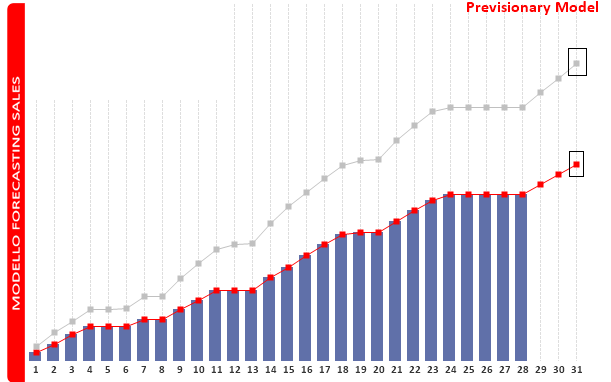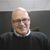# QlikView App Dev

Discussion Board for collaboration related to QlikView App Development.

Announcements
Skip the ticket, Chat with Qlik Support instead for instant assistance.
cancel
Showing results for
Did you mean:Not applicable

## Values in a variables

Dear all,

Does anyone knows if it is possible to obtain in a variable a value calculated in a chart?

I have a tricky nexted aggr exprenssion in a chart combine with multiple calculated dimensions and I cannot put this computation directly in a variables. So, I must take this value and put in a variables.

I cannot use a macro because this value must be a mandatory parameter (in the same dashboard) of another chart (with different dimensions) and it can be changed with different selections during users analysis via access point.

Kind regards

Emanuele

5 RepliesMVP

Do you have a sample you can share to help us understand better what exactly you are trying to do?Partner - Champion III

No.

You'll probably have to duplicate your expressions (and at the same time embed your dimensions in set analysis) in a variable assignment action that is triggered whenever a user changes some particular (or any) field selections.

PeterNot applicable
Author

Dear Sunny,

I Try to semplificate my case, using one dimension and two expression.

Dimension: Day: From 1 to 31 (for example)

Exprenssion

First exp:

if(Day<Num(Day(Today()),'00'),sum( {\$<YearMonth= >} CurrentValueLiq),
(
linest_m(total aggr(if(sum( {\$<YearMonth= >} ForecastValueLiq),sum( {\$<YearMonth= >} ForecastValueLiq)),Day),Day) // Pendiente
*Day // Mes a predecir
+LINEST_B(total aggr(if(sum( {\$<YearMonth= >} ForecastValueLiq),sum( {\$<YearMonth= >} ForecastValueLiq)),Day),Day) //Intercepto)
// +LINEST_SEB(total aggr(if(sum(Liquidato),sum(Liquidato)),Day),Day))

))/1000000

Second exp:

if(Day<Num(Day(Today()),'00'),sum( {\$<YearMonth= >} CurrentValueCar),
(
linest_m(total aggr(if(sum( {\$<YearMonth= >}ForecastValueCar ),sum( {\$<YearMonth= >} ForecastValueCar )),Day),Day) // Pendiente
*Day // Mes a predecir
+LINEST_B(total aggr(if(sum( {\$<YearMonth= >} ForecastValueCar ),sum( {\$<YearMonth= >} ForecastValueCar )),Day),Day) //Intercepto)
// +LINEST_SEB(total aggr(if(sum(Liquidato),sum(Liquidato)),Day),Day))

))/1000000

where:

CurrentValueLiq: is a value day by day from the first day of the month to the last day with a consolidated value

CurrentValueCar: is a value day by day from the first day of the month to the last day with a consolidated value

ForecastValueLiq: is a previsionary value from 1 to 31;

ForecastValueCar: is a previsionary value from 1 to 31;

With this expression, I obtain the chart below:I want to catch only the two last value and put it in a variables. In your opinion Is it possible?

Kind Regards

EmanuelePartner - Champion III

It is possible to make a variable that contains your "tricky nested aggr". What you will have to do is to further develop the expression that you have in the variable so it also includes the same logic that your dimensions does in the chart. To do that you will have to use Set Expressions and possibly \$-sign expansions and maybe parameters like \$1, \$2. A variable in QlikView is able to act as a function due to parameters and parameter substitution.Not applicable
Author

Dear Petter,

Yes, I found a solution.

In this case I wrote one aggr more  ( on Day ) in the variable using the expression That I wrote previously in my post , and It Seems correct.

Then I'll try with the " tricky" ones , but I think That there will be no problem , as you said

Thanks a lot

EmanueleCommunity Browser